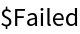Function Repository Resource:

CoordinateVector

Find the coordinate vector of a vector with respect to a basis

Contributed by: Dennis M Schneider
 ResourceFunction["CoordinateVector"][vec,basis] finds the coordinates of the vector vec with respect to the basis basis. ResourceFunction["CoordinateVector"][vec,basis,var] finds the coordinates of a vector when the vector and the basis vectors are members of a function space consisting of functions of var.

Details and Options

The input vector is given in terms of the standard basis.
The input vector may be a list of vectors.

Examples

Basic Examples (1)

Get the coordinates of a given vector in a given basis:

 In:=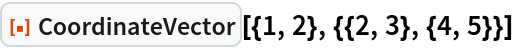Out=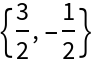Scope (15)

Vectors in ℝn

When 1 is viewed as a vector space, its elements are singletons, i.e. (α). The standard basis for 1 is {(1)}, so to find the coordinates of the vector (5) with respect to the basis {(1)}, proceed as follows:

 In:=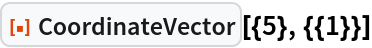Out=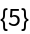Find the coordinates of a vector in 4 with respect to a basis of 4:

 In:=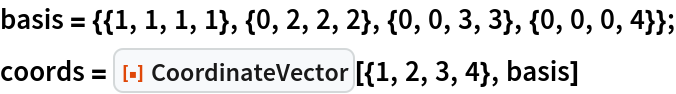Out=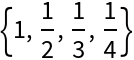Verify the result:

 In:=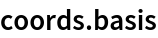Out=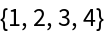Find the coordinates of a vector in 4 with respect to a basis for a three-dimensional subspace of 4:

 In:=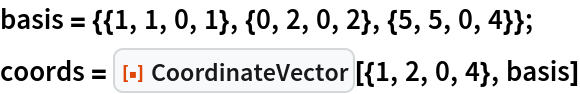Out=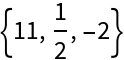Verify the result:

 In:=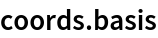Out=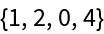Find the coordinates of a list of vectors with respect to a basis:

 In:=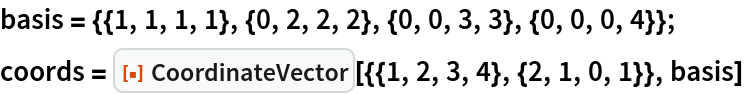Out=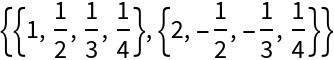Verify the result:

 In:=Out=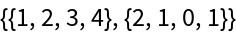Find the coordinate vector of a vector with symbolic entries:

 In:=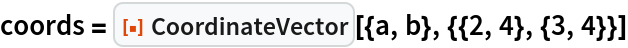Out=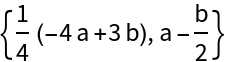Verify the result:

 In:=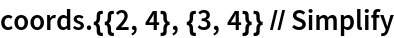Out=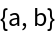If the vectors listed as the basis vectors are not independent, an error message is returned:

 In:=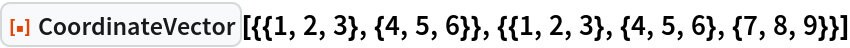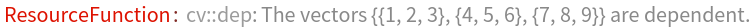Out=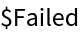Check that the given list of vectors is not independent:

 In:=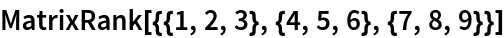Out=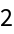If a vector is not in the span of the given list of vectors, an error message is returned:

 In:=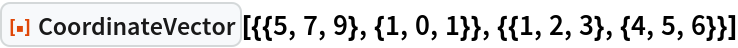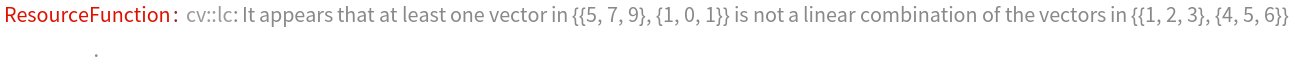Out=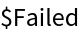Check that at least one of the given vectors is not in the span:

 In:=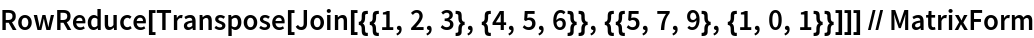Out=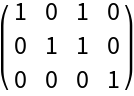Spaces of matrices (2)

The standard basis for the space of 2×4 matrices is:

 In:=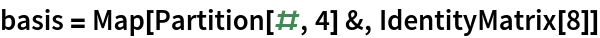Out=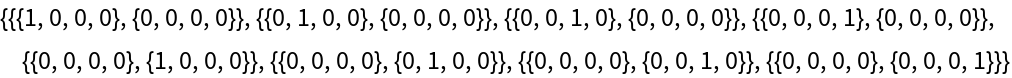Find the coordinate vector of the 2×4 matrix with respect to this basis:

 In:=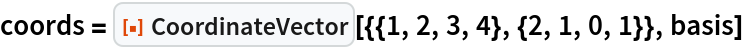Out=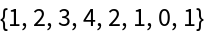Verify the result:

 In:=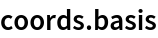Out=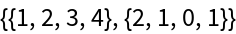Find the coordinates of a matrix with symbolic entries:

 In:=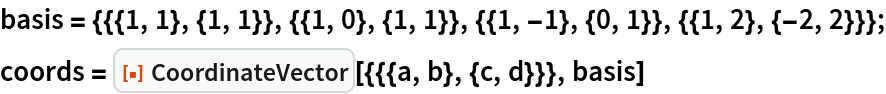Out=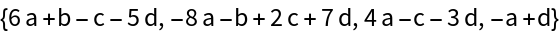Check that the result is correct:

 In:=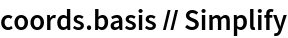Out=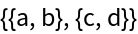Function spaces (6)

Find the coordinates of a list of two vectors (functions) with respect to the standard basis {1,x,x2,x3} of the space of polynomials of degree at most 3:

 In:=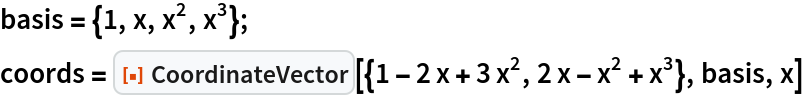Out=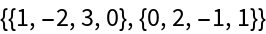Verify the result:

 In:=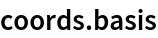Out=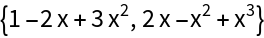Find the coordinates of two vectors (functions) with respect to a basis for a two-dimensional subspace of the space of polynomials of degree at most 4:

 In:=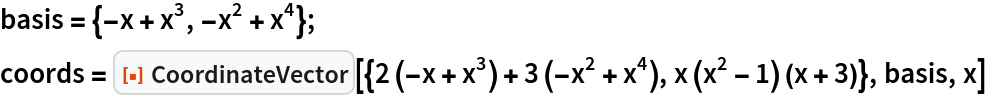Out=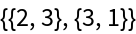Verify the result:

 In:=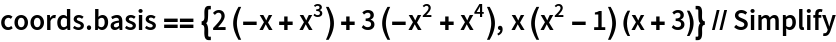Out=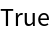This example exploits trigonometric identities to find the vector's coordinates:

 In:=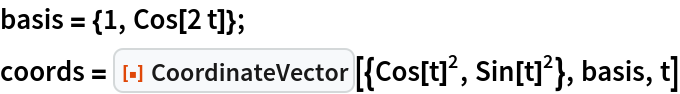Out=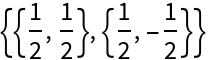Verify the result:

 In:=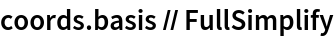Out=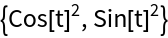Example involving exponential and hyperbolic functions:

 In:=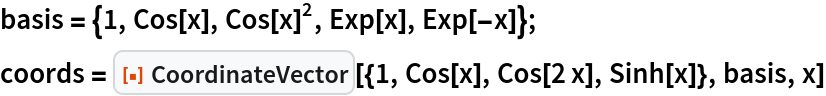Out=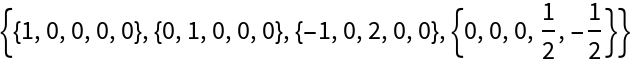Verify the result:

 In:=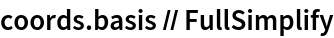Out=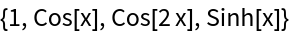A more complicated function:

 In:=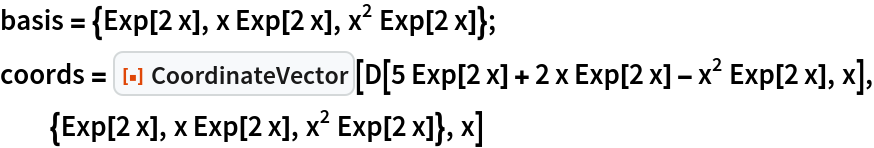Out=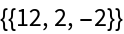Verify the result:

 In:=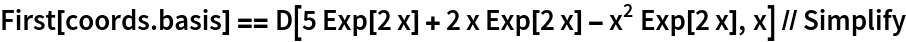Out=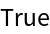The following example involves a dependent list of exponential and hyperbolic functions. The given list of vectors is dependent because the vector cos2(x) is a linear combination of 1 and cos(2x):

 In:=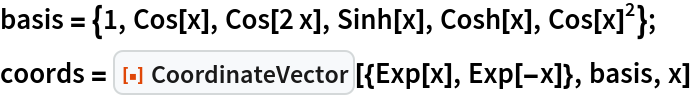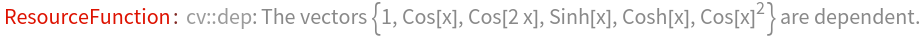Out=